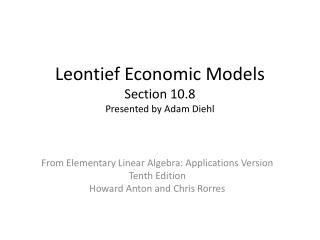# Leontief Economic Models Section 10.8 Presented by Adam Diehl - PowerPoint PPT PresentationDownload PresentationLeontief Economic Models Section 10.8 Presented by Adam Diehl

Leontief Economic Models Section 10.8 Presented by Adam DiehlDownload Presentation## Leontief Economic Models Section 10.8 Presented by Adam Diehl

- - - - - - - - - - - - - - - - - - - - - - - - - - - E N D - - - - - - - - - - - - - - - - - - - - - - - - - - -
##### Presentation Transcript

1. Leontief Economic ModelsSection 10.8Presented by Adam Diehl From Elementary Linear Algebra: Applications VersionTenth EditionHoward Anton and Chris Rorres

2. Wassilly Leontief Nobel Prize in Economics 1973. Taught economics at Harvard and New York University.

3. Economic Systems • Closed or Input/Output Model • Closed system of industries • Output of each industry is consumed by industries in the model • Open or Production Model • Incorporates outside demand • Some of the output of each industry is used by other industries in the model and some is left over to satisfy outside demand

4. Input-Output Model • Example 1 (Anton page 582)

5. Example 1 Continued p1 = daily wages of carpenter p2 = daily wages of electrician p3 = daily wages of plumber Each homeowner should receive that same value in labor that they provide.

6. Solution

7. Matrices Exchange matrix Price vector Find p such that

8. Conditions Nonnegative entries and column sums of 1 for E.

9. Key Results This equation has nontrivial solutions if Shown to always be true in Exercise 7.

10. THEOREM 10.8.1 If E is an exchange matrix, then always has a nontrivial solution pwhose entries are nonnegative.

11. THEOREM 10.8.2 Let E be an exchange matrix such that for some positive integer m all the entries of Em are positive. Then there is exactly one linearly independent solution to , and it may be chosen so that all its entries are positive. For proof see Theorem 10.5.4 for Markov chains.

12. Production Model • The output of each industry is not completely consumed by the industries in the model • Some excess remains to meet outside demand

13. Matrices Production vector Demand vector Consumption matrix

14. Conditions Nonnegative entries in all matrices.

15. Consumption Row i (i=1,2,…,k) is the amount of industry i’s output consumed in the production process.

16. Surplus Excess production available to satisfy demand is given by C and d are given and we must find x to satisfy the equation.

17. Example 5 (Anton page 586) • Three Industries • Coal-mining • Power-generating • Railroad x1 = \$ output coal-mining x2 = \$ output power-generating x3 = \$ output railroad

18. Example 5 Continued

19. Solution

20. Productive Consumption Matrix If is invertible, If all entries of are nonnegative there is a unique nonnegative solution x. Definition: A consumption matrix C is said to be productive if exists and all entries of are nonnegative.

21. THEOREM 10.8.3 A consumption matrix C is productive if and only if there is some production vector x  0 such thatx Cx. For proof see Exercise 9.

22. COROLLARY 10.8.4 A consumption matrix is productive if each of its row sums is less than 1.

23. COROLLARY 10.8.5 A consumption matrix is productive if each of its column sums is less than 1. (Profitable consumption matrix) For proof see Exercise 8.